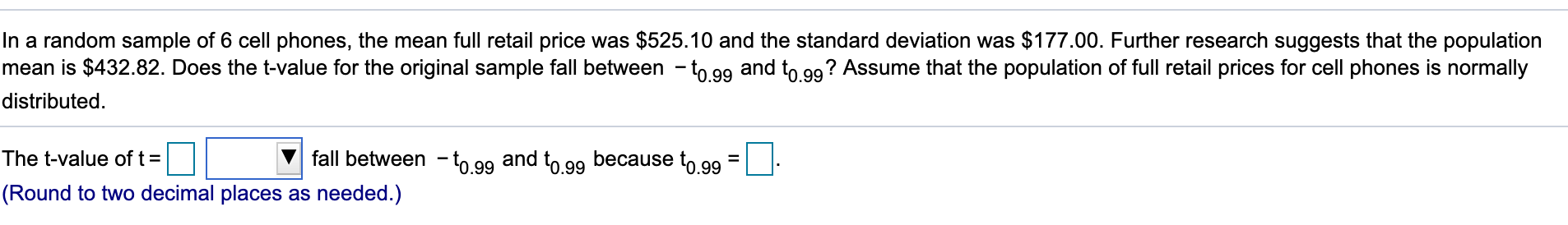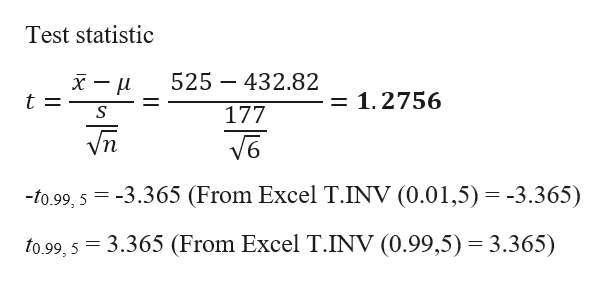# In a random sample of 6 cell phones, the mean full retail price was \$525.10 and the standard deviation was \$177.00. Further research suggests that the populationmean is \$432.82. Does the t-value for the original sample fall between -to gg and to gg? Assume that the population of full retail prices for cell phones is normallydistributedThe t-value of tfall between to g9 and to gg because to g9 =.990.99(Round to two decimal placess as needed.)

Question
128 views

I'm not sure what t-value is in order so solve for it.help_outlineImage TranscriptioncloseIn a random sample of 6 cell phones, the mean full retail price was \$525.10 and the standard deviation was \$177.00. Further research suggests that the population mean is \$432.82. Does the t-value for the original sample fall between -to gg and to gg? Assume that the population of full retail prices for cell phones is normally distributed The t-value of t fall between to g9 and to gg because to g9 = .99 0.99 (Round to two decimal placess as needed.) fullscreen
check_circle

Step 1

The t-distribution is used, because the population standard deviation is unknown and the sample standard deviation is being used as a substitute.

Calculation:

Here, n = 6;  = 525.10; s = 177.00.

There...help_outlineImage TranscriptioncloseTest statistic 525 432.82 = 1.2756 t 177 Vn -to.99, 5-3.365 (From Excel T.INV (0.01,5) -3.365) to.99, 5 3.365 (From Excel T.INV (0.99,5) 3.365) fullscreen

### Want to see the full answer?

See Solution

#### Want to see this answer and more?

Solutions are written by subject experts who are available 24/7. Questions are typically answered within 1 hour.*

See Solution
*Response times may vary by subject and question.
Tagged in

### Hypothesis Testing